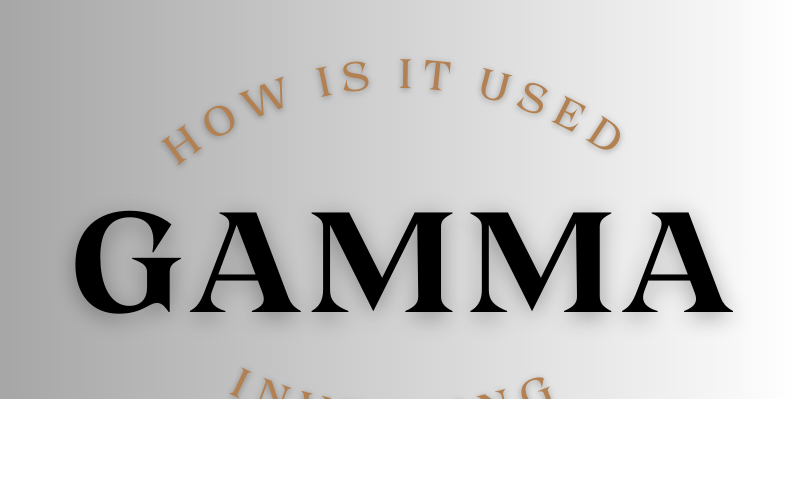Connect with us

Hi, what are you looking for?

DOGE0.070.84%SOL19.370.72%USDC1.000.01%BNB287.900.44%AVAX15.990.06%XLM0.080.37%

# Gamma in Investing and How Is It Used?File Photo: Gamma in Investing and How Is It Used? File Photo: Gamma in Investing and How Is It Used?

### What’s Gamma in Investing?

Gamma (Γ) measures options risk by measuring the delta change per one-point change in the underlying asset’s price. Delta measures how much an option’s premium (price) changes with a one-point asset price change. Thus, gamma measures how an option’s price changes with the underlying price. Option prices are more volatile with higher gamma.

Gamma measures the convexity of a derivative’s value relative to the underlying asset. It is one of the “options Greeks,” along with delta, rho, theta, and vega. These evaluate option portfolio risk.

### Understanding Gamma in Investing

Gamma evaluates option price movement relative to in-the-money or out-of-the-money amounts. It is the first derivative of delta. It shows how the delta varies with the asset. If the gamma is ten and the delta is +40, a \$1 increase in the underlying price would make the delta +50.

Gamma is low for deep in- or out-of-the-money options; it is also highest when the option is near or at the money. Gamma is also higher for near-term options compared to extended options.

A crucial measure addresses convexity difficulties in option hedging methods. Some portfolio managers or traders handle enormous portfolios that require more precision when hedging. Use “color” as a third-order derivative. The color indicates gamma change and is crucial for gamma-hedged portfolios.

Like physics, an option’s delta is its “speed,” and its gamma is its “acceleration.”

### Uses of Gamma in Investing

Gamma gives traders a more accurate view of how an option’s delta will evolve as the underlying price changes, while delta measures are only relevant for a limited time. Delta measures the option price’s response to underlying asset price fluctuations.

Delta approaches one, and gamma drops toward zero when an option moves further in the money. Gamma approaches 0 when an option moves out of the money—gamma peaks at the money price.

Formulating gamma is complex and involves financial software or spreadsheets. This is an estimated gamma calculation. Consider a call option on a stock with a delta of 0.40. The option’s delta and value will increase by 40 cents if the stock rises by \$1.00. After the \$1 rise, assume the option delta is 0.53. The delta difference of 0.13 approximates gamma.

All extended options have a positive gamma, whereas all short options have a negative one.

### Example of Gamma in Investing

Consider a stock trading at \$10 with a delta of 0.5 and a gamma of 0.10. The delta will adjust by 0.10 for every \$1 stock price change. A \$1.00 rise raises the option’s differential to 0.60. Delta drops to 0.40 for every \$1.00 reduction.

### How can traders hedge Gamma?

Gamma hedging aims to maintain a consistent delta in an options position. They buy and sell options to offset each other, resulting in a net gamma of near zero. At this moment, the posture is considered gamma-neutral. Many traders want to maintain zero gammas around a delta-neutral position. Use delta-gamma hedging, where net delta and net gamma are near zero. This protects an option’s value against asset price movements.

### A long-gamma strategy?

Long-gamma traders’ options delta rises with asset prices. As the price increases, a long gamma position will show a growing delta or, as it falls, a decreasing delta. Long-gamma exposure can help traders profit by encouraging them to purchase cheaply and sell high.

### Is gamma risky?

Short-gamma options risk compounded losses due to underlying price changes. If a delta-neutral position increases as the stock does, the deltas will shorten, causing the options to lose more money. However, buying deltas at these higher prices might cause the underlying asset to plummet, producing long deltas on the way down and compounding prior losses.

### Conclusion

Gamma quantifies delta change per one-point asset growth. It helps traders predict option deltas or position changes. Gamma increases for at-the-money options and decreases for in- and out-of-the-money options. Unlike delta, gamma always favors long calls and puts.

Investopedia does not offer financial, investing, or tax advice. The material may not be suitable for all investors since it does not consider their investing goals, risk tolerance, or financial situation. Investments include risk, including principal loss.

### Conclusion

• Based on a single-point price change, gamma is an option’s delta rate of change.
• It is a second-order risk factor or delta of the delta.
• Gamma is highest at the money and lowest away from it.
• All else being equal, options near expiration have the greatest gamma.
• Gamma measures how asset changes impact option moneyness.
• Delta-gamma hedging protects options against asset movements.